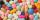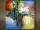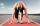Diplomas

Suzanne helped write out diplomas. Issuance its first took 3 minutes, each next 2.3 minutes. How much time it will take to write out n=16 diplomas (including the first), write out this pace?

Result

x =  37.5 min

Solution:Leave us a comment of this math problem and its solution (i.e. if it is still somewhat unclear...):Be the first to comment!Next similar examples:

1. Days2minutesWrite a formula that converts x days to y minutes. The formula is:
2. PillsIf it takes 20 minutes to run a batch of 100 pills how many minutes would it take to run a batch of 50 pills
3. Masons1 mason casts 30.8 meters square in 8 hours. How long casts 4 masons 178 meters square?
4. Image scaleThe actual image dimensions are 60 cm x 80cm and has a reduced size 3 cm x 4 cm. At what scale the image was reduced?
5. DonutsFind how many donuts each student will receive if you share 126 donuts in a ratio of 1:5:8
6. Greg and BillGreg is 18 years old. He is 6 less than 4 times Bill's age. How old is Bill?
7. Grandfather and grandmotherThe old mother is 5 years younger than the old father. Together they are 153 years old. How many years has each of them?
8. TVsProduction of television sets increased from 3,500 units to 4,200 units. Calculate the percentage of production increase.
9. Scale of the mapDetermine the scale of the map if the actual distance between A and B is 720 km and distance on the map is 20 cm.
10. Unknown numberIdentify unknown number which 1/5 is 40 greater than one tenth of that number.
11. ClassIn a class are 32 pupils. Of these are 8 boys. What percentage of girls are in the class?
12. Conference148 is the total number of employees. The conference was attended by 22 employees. How much is it in percent?
13. Percents - easyHow many percent is 432 out of 434?
14. Runner 2Marian run 12 meters in 8 seconds. How far would Marian run for 70 seconds, if still run at the same pace?
15. GlovesI have a box with two hundred pieces of gloves in total, split into ten parcels of twenty pieces, and I sell three parcels. What percent of the total amount I sold?
16. Down syndromeDown syndrome is one of the serious diseases caused by a gene mutation. Down syndrome occurs in approximately every 550-born child. Express the incidence of Down's syndrome in newborns at per mille.
17. Apples 2James has 13 apples. He has 30 percent more apples than Sam. How many apples has Sam?